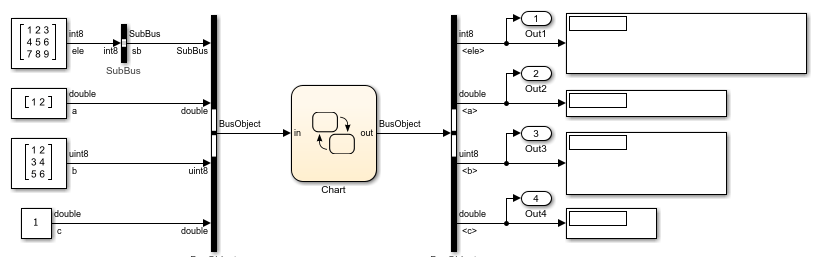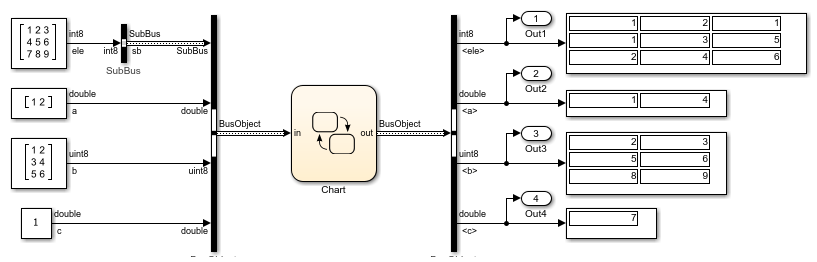# Index and Assign Values to Stateflow Structures

This example shows how to access and modify the contents of a Stateflow® structure or an array of Stateflow structures. A Stateflow structure is a data type that you define from a `Simulink.Bus (Simulink)` object. You can use Stateflow structures to bundle data of different sizes and types together into a single data object. For more information, see Access Bus Signals Through Stateflow Structures.

### Index Substructures and Fields

To index substructures and fields of Stateflow structures, use dot notation. The first part of a name identifies the parent structure. Subsequent parts identify the children along a hierarchical path. The children can be individual fields or fields that contain other structures (also called substructures). The names of the fields of a Stateflow structure match the names of the elements of the `Simulink.Bus` object that defines the structure. When a field contains a vector, matrix, or array, you can access its elements by using the indexing notation supported by the action language of your chart.

For example, the chart in this model contains an input structure (`in`), an output structure (`out`), a local structure (`localbus`), and a local array of structures (`subBusArray`).

• The chart defines the input structure `in`, the output structure `out`, and the local structure `localbus` by using the `Simulink.Bus` object `BusObject`. These structures have four fields: `sb`, `a`, `b`, and `c`.

• The field `sb` is a substructure defined from the `Simulink.Bus` object `SubBus`. This substructure has one field called `ele`.

• The chart defines the local array of structures `subBusArray` by using the `Simulink.Bus` object `SubBus`. The array has size 4. Each element in the array is a structure with one field called `ele`.This list illustrates expressions that combine dot notation and numeric indices based on the structure specifications for this example:

• `in.c` — Field `c` of the input structure `in`

• `in.a(1)` — First element of the vector field `a` of the input structure `in`

• `out.sb` — Substructure `sb` of the output structure `out`

• `out.sb.ele` — Field `ele` of the substructure `out.sb`

• `out.sb.ele(2,2)` — Element in the second row, second column of the field `ele` of the substructure `out.sb`

• `subBusArray(1)` — First element of the array of structures `subBusArray`

• `subBusArray(1).ele` — Field `ele` of the structure `subBusArray(1)`

• `subBusArray(1).ele(3,4)` — Element in the third row, fourth column of the field `ele` of structure `subBusArray(1)`

Because the chart uses MATLAB as the action language, you access the elements of the arrays in this example by using one-based indexing delimited by parentheses. In charts that use C as the action language, use zero-based indexing delimited by brackets. For more information, see Operations for Vectors and Matrices in Stateflow.

### Assign Values to Structures and Fields

You can write to any Stateflow structure that has a scope other than `Input`. You can assign values to the entire structure, to a substructure, or to a single field.

• To assign one structure to another structure, define both structures from the same `Simulink.Bus` object in the base workspace.

• To assign one structure to a substructure of a different structure (or the other way around), define the structure and substructure from the same `Simulink.Bus` object.

• To assign a field of one structure to a field of another structure, the fields must have the same type and size. You can define the Stateflow structures from different `Simulink.Bus` objects.

For instance, the chart in this example makes these assignments:

• `localbus = sb2abc(in.sb)` — The structure `localbus` and the output argument of the MATLAB® function `sb2abc` are defined from the same `Simulink.Bus` object `BusObject`. The function decomposes its input into three components: a vector, a 3-by-2 matrix, and a scalar. The function returns these components as the fields `a`, `b`, and `c` of its output. For more information on this function, see Access Simulink Bus Signals in MATLAB Functions.

• `subBusArray(1) = in.sb` — The structure `subBusArray(1)` and the substructure `in.sb` are defined from the same `Simulink.Bus` object `SubBus`.

• `subBusArray(2) = abc2sb(in)` — The structure `subBusArray(2)` and the output argument of the graphical function `abc2sb` are defined from the same `Simulink.Bus` object `SubBus`. The function combines the values of the fields `a`, `b`, and `c` from its input and rearranges them in a 3-by-3 matrix of type `int8`. It returns this matrix as the field `ele` of its output.

• `subBusArray(3).ele = transpose(in.sb.ele)` — The field `subBusArray(3).ele` has the same type and size as the result of `transpose(in.sb.ele)`. Both are 3-by-3 matrices of type `int8`.

• `subBusArray(4).ele = int8(magic(3))` — The field `subBusArray(4).ele` has the same type and size as the result of `int8(magic(3))`. Both are 3-by-3 matrices of type `int8`.

• `out = localbus` — Both `out` and `localbus` are defined from the same `Simulink.Bus` object `BusObject`.

• `out.sb = subBusArray(idx)` — The substructure `out.sb` and the structure `subBusArray(idx)` are defined from the same `Simulink.Bus` object `SubBus`.### Run the Simulation

When you simulate the example, the chart uses the values of the field `sb` of the input structure to populate the fields `a`, `b`, and `c` of the output structure. The parameter `idx` selects the element of the array of structures `subBusArray` to use as the substructure `sb` of the output. In this example, `idx` equals 2, so the chart uses the values of the fields `a`, `b`, `c` of the input structure to populate the substructure.When you use other values for `idx`, the substructure `out.sb` contains the same values as `in.sb`, the transpose of `in.sb`, or a 3-by-3 magic square.